In :
%matplotlib inline
%config InlineBackend.figure_format = 'retina'

In :
import os
import math
import warnings
warnings.filterwarnings('ignore')

from IPython.display import Image, HTML
import numpy as np
import pandas as pd
import seaborn as sns
import matplotlib.pyplot as plt
import pymc # I know folks are switching to "as pm" but I'm just not there yet

In :
DATA_DIR = os.path.join(os.getcwd(), 'data/')

In :
data_file = DATA_DIR + 'results_2014.csv'
df = pd.read_csv(data_file, sep=',')
df.head()

Out:
home_team away_team home_score away_score
0 Wales Italy 23 15
1 France England 26 24
2 Ireland Scotland 28 6
3 Ireland Wales 26 3
4 Scotland England 0 20
In :
teams = df.home_team.unique()
teams = pd.DataFrame(teams, columns=['team'])
teams['i'] = teams.index
teams.head()

Out:
team i
0 Wales 0
1 France 1
2 Ireland 2
3 Scotland 3
4 Italy 4
In :
teams

Out:
team i
0 Wales 0
1 France 1
2 Ireland 2
3 Scotland 3
4 Italy 4
5 England 5
In :
df = pd.merge(df, teams, left_on='home_team', right_on='team', how='left')
df = df.rename(columns = {'i': 'i_home'}).drop('team', 1)
df = pd.merge(df, teams, left_on='away_team', right_on='team', how='left')
df = df.rename(columns = {'i': 'i_away'}).drop('team', 1)
df.head()

Out:
home_team away_team home_score away_score i_home i_away
0 Wales Italy 23 15 0 4
1 France England 26 24 1 5
2 Ireland Scotland 28 6 2 3
3 Ireland Wales 26 3 2 0
4 Scotland England 0 20 3 5
In :
observed_home_goals = df.home_score.values
observed_away_goals = df.away_score.values
home_team = df.i_home.values
away_team = df.i_away.values
num_teams = len(df.i_home.drop_duplicates())
num_games = len(home_team)

In :
num_teams

Out:
6

The model. The league is made up by a total of T= 6 teams, playing each other once in a season. We indicate the number of points scored by the home and the away team in the g-th game of the season (15 games) as $y_{g1}$ and $y_{g2}$ respectively. The vector of observed counts $\mathbb{y} = (y_{g1}, y_{g2})$ is modelled as independent Poisson: $$y_{gi}| \theta_{gj} \tilde\;\; Poisson(\theta_{gj})$$ where the theta parameters represent the scoring intensity in the g-th game for the team playing at home (j=1) and away (j=2), respectively. We model these parameters according to a formulation that has been used widely in the statistical literature, assuming a log-linear random effect model: $$log \theta_{g1} = home + att_{h(g)} + def_{a(g)}$$ $$log \theta_{g2} = att_{a(g)} + def_{h(g)}$$ the parameter home represents the advantage for the team hosting the game and we assume that this effect is constant for all the teams and throughout the season. In addition, the scoring intensity is determined jointly by the attack and defense ability of the two teams involved, represented by the parameters att and def, respectively. In line with the Bayesian approach, we have to specify some suitable prior distributions for all the random parameters in our model. The variable $$home$$ is modelled as a fixed effect, assuming a standard flat prior distribution. We use the notation of describing the Normal distribution in terms of mean and the precision. $$home \tilde\; Normal(0,0.0001)$$ Conversely, for each t = 1, ..., T, the team-specific effects are modelled as exchangeable from a common distribution: $$att_t \tilde\; Normal(\mu_{att}, \tau_{att})$$ $$def_t \tilde\; Normal(\mu_{def}, \tau_{def})$$ In the first model from the paper, they model the observed goals-scored counts as:

"where the represent the scoring intensity in the g-th game for the team playing at home (j = 1) and away (j = 2), respectively." They use a log-linear model for the thetas:

Note that they're breaking out team strength into attacking and defending strength. A negative defense parameter will sap the mojo from the opposing team's attacking parameter.

home represents home-team advantage, and in this model is assumed to be constant across teams. The prior on the home and intercept parameters is flat (keep in mind that in pymc, Normals are specified as (mean, precision):

The team-specific effects are modeled as exchangeable:

To ensure identifiability, they impose a sum-to-zero constraint on the attack and defense parameters. They also tried a corner constraint (pinning one team's parameters to 0,0), but found that interpretation is easier in the sum-to-zero case because it's not relative to the 0,0 team.

The hyper-priors on the attack and defense parameters are also flat.

I made two tweaks to the model. It didn't make sense to me to have a μatt when we're enforcing the sum-to-zero constraint by subtracting the mean anyway. So I eliminated $$\mu_{att}$$ and $$\mu_{def}$$

Also because of the sum-to-zero constraint, it seemed to me that we needed an intercept term in the log-linear model, capturing the average goals scored per game by the away team. This we model with the following hyperprior. $$intercept \tilde\; Normal(0, 0.001)$$

In :
df

Out:
home_team away_team home_score away_score i_home i_away
0 Wales Italy 23 15 0 4
1 France England 26 24 1 5
2 Ireland Scotland 28 6 2 3
3 Ireland Wales 26 3 2 0
4 Scotland England 0 20 3 5
5 France Italy 30 10 1 4
6 Wales France 27 6 0 1
7 Italy Scotland 20 21 4 3
8 England Ireland 13 10 5 2
9 Ireland Italy 46 7 2 4
10 Scotland France 17 19 3 1
11 England Wales 29 18 5 0
12 Italy England 11 52 4 5
13 Wales Scotland 51 3 0 3
14 France Ireland 20 22 1 2
In :
g = df.groupby('i_away')
att_starting_points = np.log(g.away_score.mean())
g = df.groupby('i_home')
def_starting_points = -np.log(g.away_score.mean())

In :
#hyperpriors
home = pymc.Normal('home', 0, .0001, value=0)
tau_att = pymc.Gamma('tau_att', .1, .1, value=10)
tau_def = pymc.Gamma('tau_def', .1, .1, value=10)
intercept = pymc.Normal('intercept', 0, .0001, value=0)

In :
#team-specific parameters
atts_star = pymc.Normal("atts_star",
mu=0,
tau=tau_att,
size=num_teams,
value=att_starting_points.values)
defs_star = pymc.Normal("defs_star",
mu=0,
tau=tau_def,
size=num_teams,
value=def_starting_points.values)

In :
# trick to code the sum to zero contraint
@pymc.deterministic
def atts(atts_star=atts_star):
atts = atts_star.copy()
atts = atts - np.mean(atts_star)
return atts

@pymc.deterministic
def defs(defs_star=defs_star):
defs = defs_star.copy()
defs = defs - np.mean(defs_star)
return defs

@pymc.deterministic
def home_theta(home_team=home_team,
away_team=away_team,
home=home,
atts=atts,
defs=defs,
intercept=intercept):
return np.exp(intercept +
home +
atts[home_team] +
defs[away_team])

@pymc.deterministic
def away_theta(home_team=home_team,
away_team=away_team,
home=home,
atts=atts,
defs=defs,
intercept=intercept):
return np.exp(intercept +
atts[away_team] +
defs[home_team])

home_points = pymc.Poisson('home_points',
mu=home_theta,
value=observed_home_goals,
observed=True)
away_points = pymc.Poisson('away_points',
mu=away_theta,
value=observed_away_goals,
observed=True)

mcmc = pymc.MCMC([home, intercept, tau_att, tau_def,
home_theta, away_theta,
atts_star, defs_star, atts, defs,
home_points, away_points])
map_ = pymc.MAP( mcmc )
map_.fit()
mcmc.sample(200000, 40000, 20)

 [-----------------100%-----------------] 200000 of 200000 complete in 390.2 sec
In :
pymc.Matplot.plot(home)

Plotting home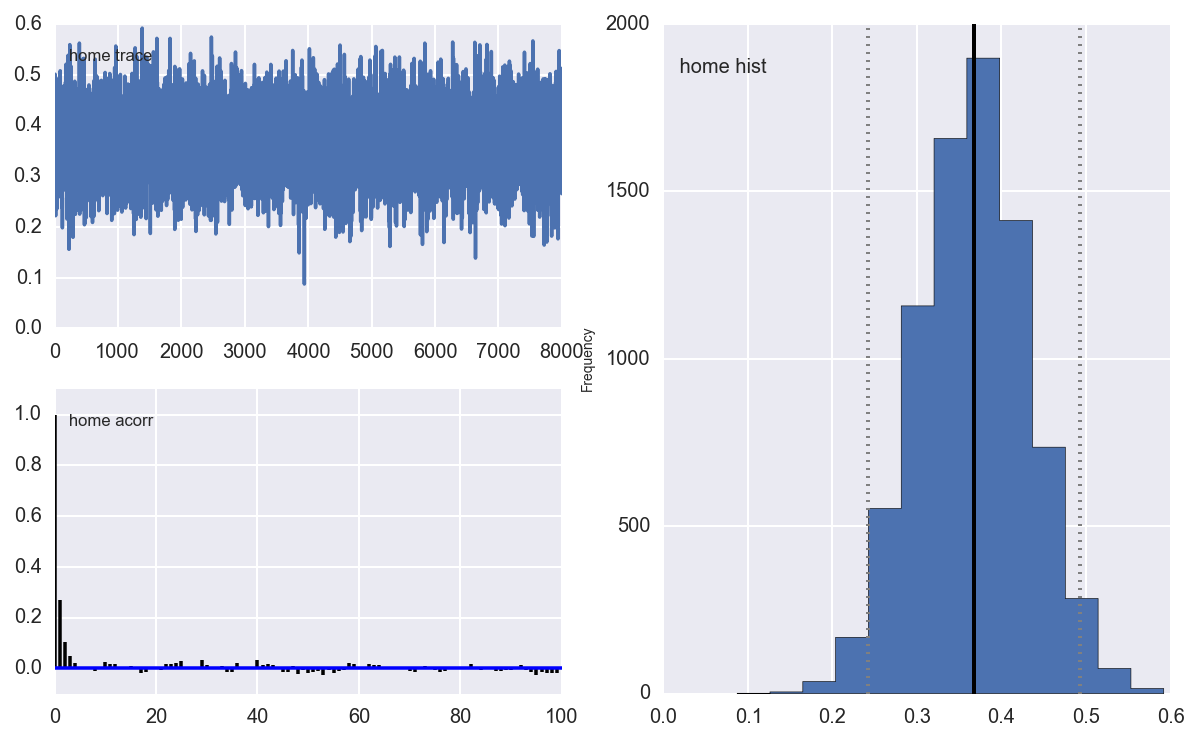In :
pymc.Matplot.plot(intercept)

Plotting intercept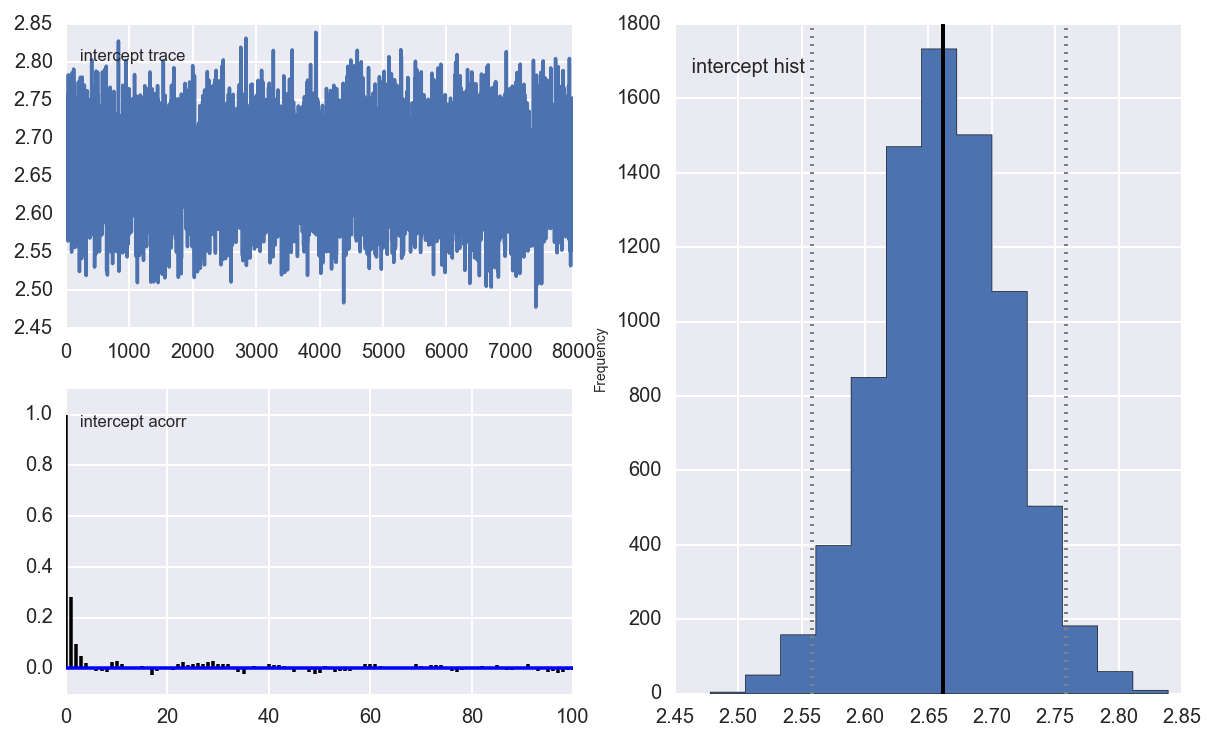In :
pymc.Matplot.plot(tau_att)

Plotting tau_att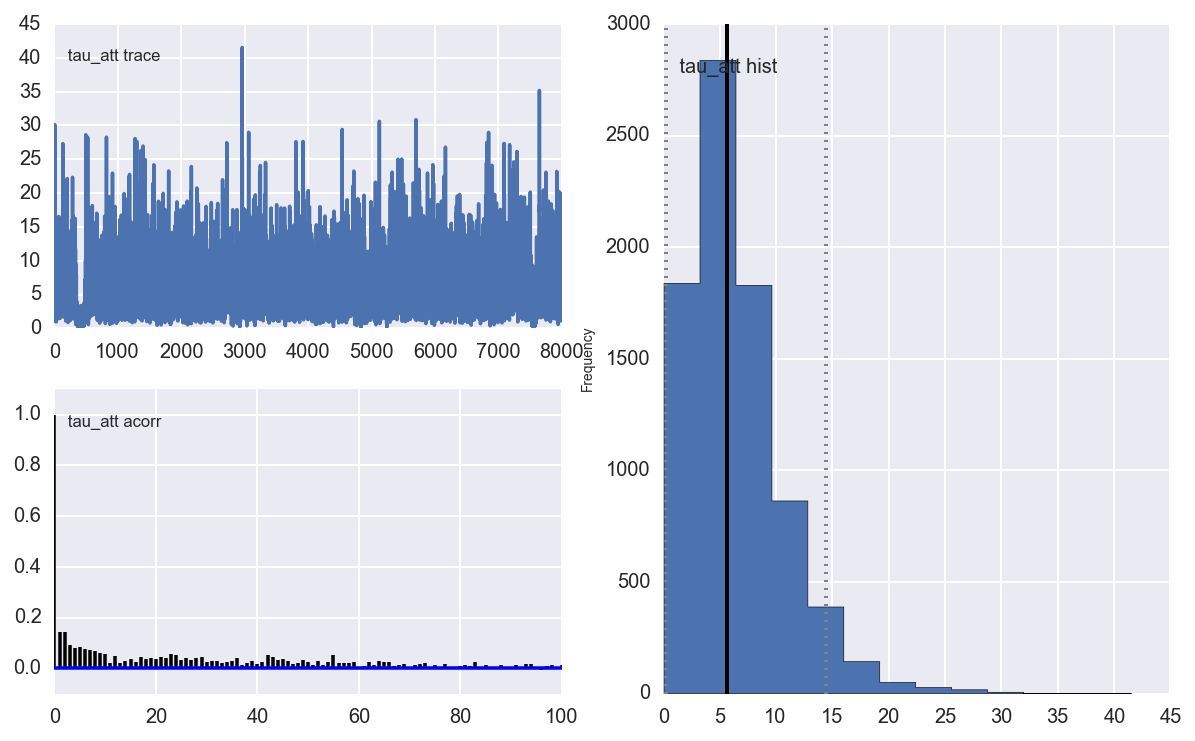In :
pymc.Matplot.plot(tau_def)

Plotting tau_def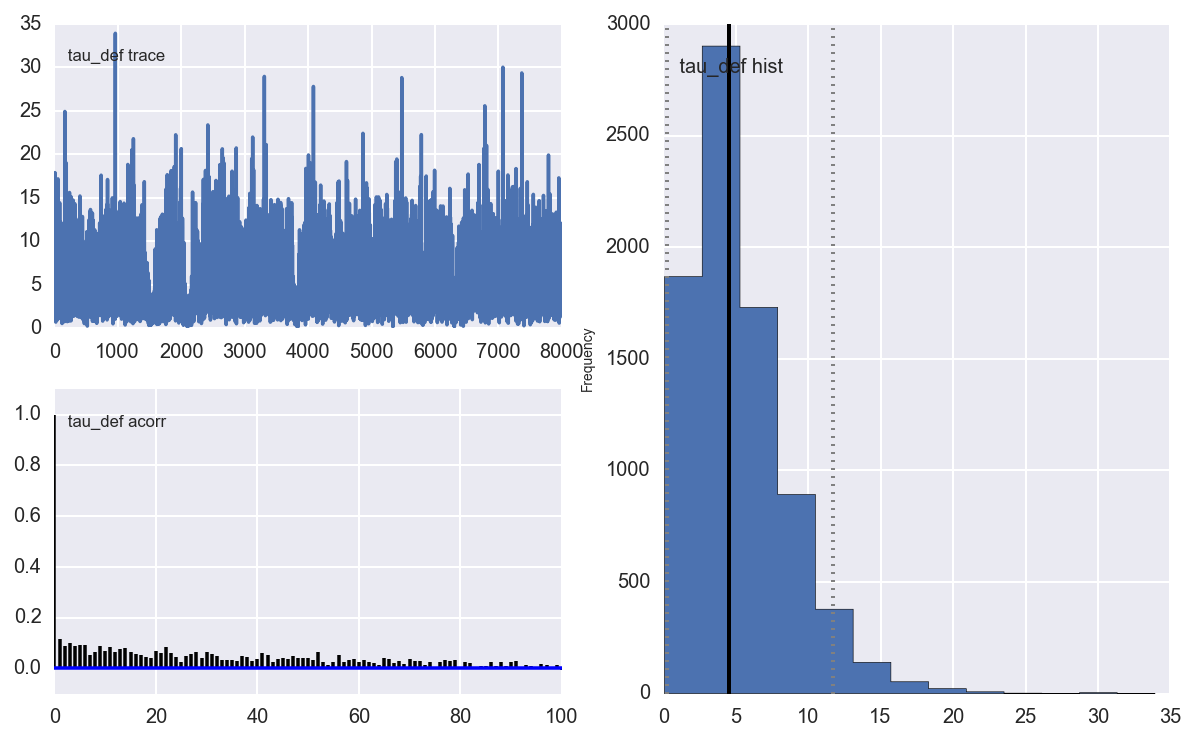In :
observed_season = DATA_DIR + 'table_2014.csv'
df_observed = pd.read_csv(observed_season)
df_observed.loc[df_observed.QR.isnull(), 'QR'] = ''

In :
def simulate_season():
"""
Simulate a season once, using one random draw from the mcmc chain.
"""
num_samples = atts.trace().shape
draw = np.random.randint(0, num_samples)
atts_draw = pd.DataFrame({'att': atts.trace()[draw, :],})
defs_draw = pd.DataFrame({'def': defs.trace()[draw, :],})
home_draw = home.trace()[draw]
intercept_draw = intercept.trace()[draw]
season = df.copy()
season = pd.merge(season, atts_draw, left_on='i_home', right_index=True)
season = pd.merge(season, defs_draw, left_on='i_home', right_index=True)
season = season.rename(columns = {'att': 'att_home', 'def': 'def_home'})
season = pd.merge(season, atts_draw, left_on='i_away', right_index=True)
season = pd.merge(season, defs_draw, left_on='i_away', right_index=True)
season = season.rename(columns = {'att': 'att_away', 'def': 'def_away'})
season['home'] = home_draw
season['intercept'] = intercept_draw
season['home_theta'] = season.apply(lambda x: math.exp(x['intercept'] +
x['home'] +
x['att_home'] +
x['def_away']), axis=1)
season['away_theta'] = season.apply(lambda x: math.exp(x['intercept'] +
x['att_away'] +
x['def_home']), axis=1)
season['home_goals'] = season.apply(lambda x: np.random.poisson(x['home_theta']), axis=1)
season['away_goals'] = season.apply(lambda x: np.random.poisson(x['away_theta']), axis=1)
season['home_outcome'] = season.apply(lambda x: 'win' if x['home_goals'] > x['away_goals'] else
'loss' if x['home_goals'] < x['away_goals'] else 'draw', axis=1)
season['away_outcome'] = season.apply(lambda x: 'win' if x['home_goals'] < x['away_goals'] else
'loss' if x['home_goals'] > x['away_goals'] else 'draw', axis=1)
season = season.join(pd.get_dummies(season.home_outcome, prefix='home'))
season = season.join(pd.get_dummies(season.away_outcome, prefix='away'))
return season

def create_season_table(season):
"""
Using a season dataframe output by simulate_season(), create a summary dataframe with wins, losses, goals for, etc.

"""
g = season.groupby('i_home')
home = pd.DataFrame({'home_goals': g.home_goals.sum(),
'home_goals_against': g.away_goals.sum(),
'home_wins': g.home_win.sum(),
'home_losses': g.home_loss.sum()
})
g = season.groupby('i_away')
away = pd.DataFrame({'away_goals': g.away_goals.sum(),
'away_goals_against': g.home_goals.sum(),
'away_wins': g.away_win.sum(),
'away_losses': g.away_loss.sum()
})
df = home.join(away)
df['wins'] = df.home_wins + df.away_wins
df['losses'] = df.home_losses + df.away_losses
df['points'] = df.wins * 2
df['gf'] = df.home_goals + df.away_goals
df['ga'] = df.home_goals_against + df.away_goals_against
df['gd'] = df.gf - df.ga
df = pd.merge(teams, df, left_on='i', right_index=True)
df = df.sort_index(by='points', ascending=False)
df = df.reset_index()
df['position'] = df.index + 1
df['champion'] = (df.position == 1).astype(int)
df['relegated'] = (df.position > 5).astype(int)
return df

def simulate_seasons(n=100):
dfs = []
for i in range(n):
s = simulate_season()
t = create_season_table(s)
t['iteration'] = i
dfs.append(t)
return pd.concat(dfs, ignore_index=True)

In [ ]:
simuls = simulate_seasons(10000)

In :
ax = simuls.points[simuls.team == 'Ireland'].hist(figsize=(7,5))
median = simuls.points[simuls.team == 'Ireland'].median()
ax.set_title('Ireland: 2013-14 points, 1000 simulations')
ax.plot([median, median], ax.get_ylim())
plt.annotate('Median: %s' % median, xy=(median + 1, ax.get_ylim()-10))

Out:
<matplotlib.text.Annotation at 0xa93f00ec>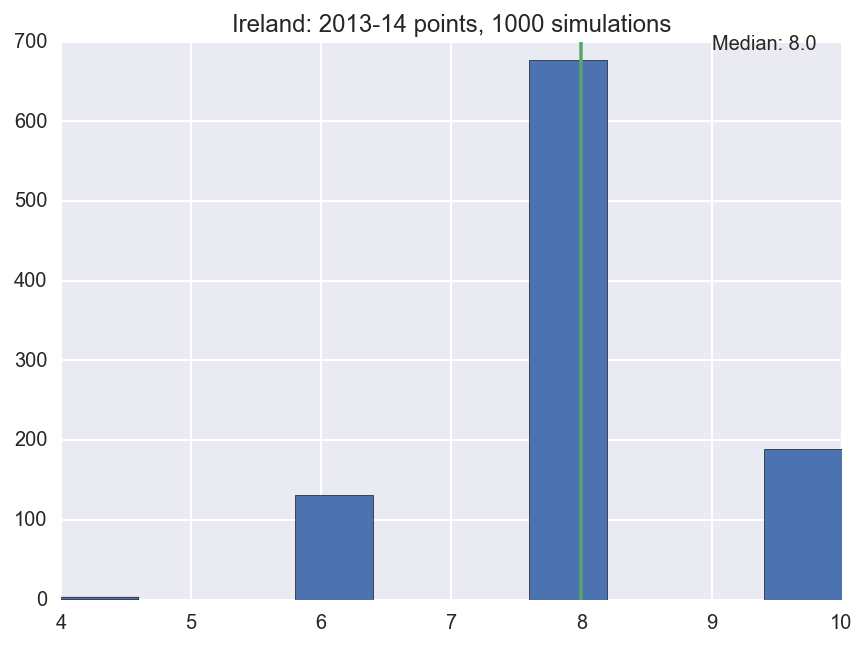In :
ax = simuls.gf[simuls.team == 'Ireland'].hist(figsize=(7,5))
median = simuls.gf[simuls.team == 'Ireland'].median()
ax.set_title('Ireland: 2013-14 points for, 1000 simulations')
ax.plot([median, median], ax.get_ylim())
plt.annotate('Median: %s' % median, xy=(median + 1, ax.get_ylim()-10))

Out:
<matplotlib.text.Annotation at 0xa933546c>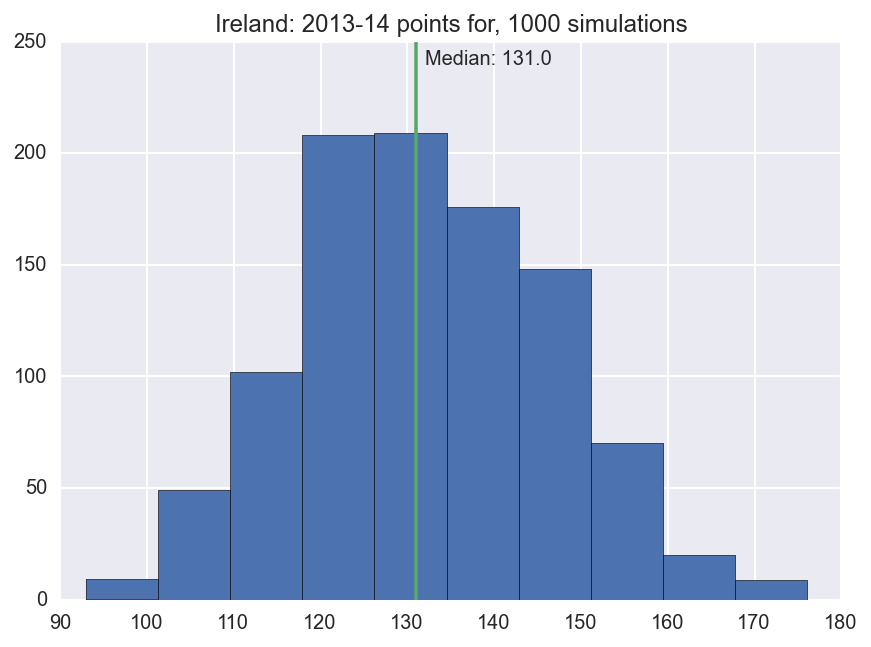In :
g = simuls.groupby('team')
df_champs = pd.DataFrame({'percent_champs': g.champion.mean()})
df_champs = df_champs.sort_index(by='percent_champs')
df_champs = df_champs[df_champs.percent_champs > .05]
df_champs = df_champs.reset_index()

fig, ax = plt.subplots(figsize=(8,6))
ax.barh(df_champs.index.values, df_champs.percent_champs.values)

for i, row in df_champs.iterrows():
label = "{0:.1f}%".format(100 * row['percent_champs'])
ax.annotate(label, xy=(row['percent_champs'], i), xytext = (3, 10), textcoords = 'offset points')
ax.set_ylabel('Team')
ax.set_title('% of Simulated Seasons In Which Team Finished Winners')
_= ax.set_yticks(df_champs.index + .5)
_= ax.set_yticklabels(df_champs['team'].values)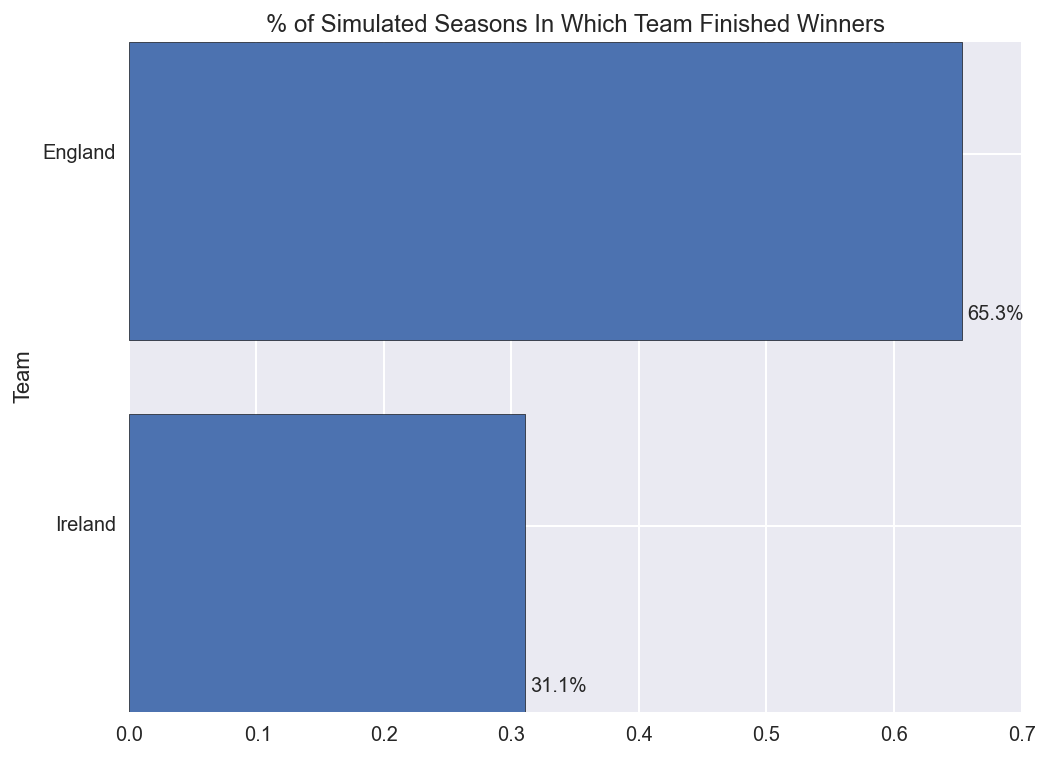In [ ]: